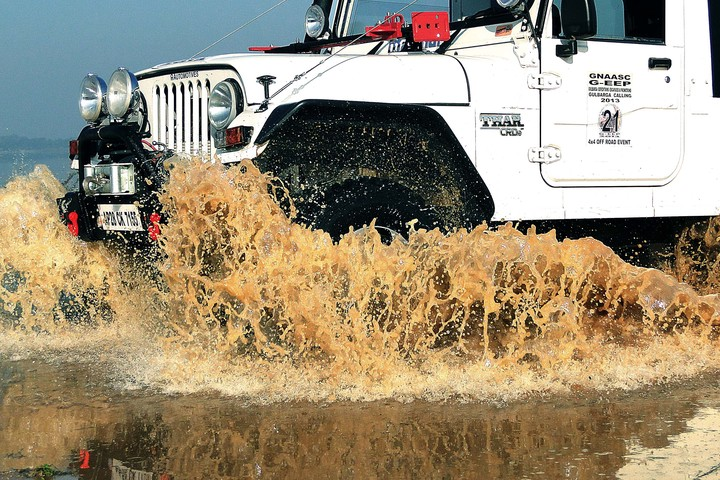Other optional upgrades for the TharNote All prices are ex-Hyderabad. In case of out-of-station orders, packing, forwarding & installation charges will be extra as per the area of delivery.

1. Fiber Snorkel setup
₹ 14,000.00 + 18% GST (2,520.00) = Total ₹ 16,250.00

2. External Roll Cage (compatible with low roof Hard Top)
₹ 24,000.00 + 18% GST (4,320.00) = Total ₹ 28,320.00

3. Front Bumper with winch plate, Bull bar
₹ 15,000.00 + 18% GST (2,700.00) = Total ₹ 17,700.00

4. Rear Bumper with Stepny Bracket & Jerry Can holder
₹ 18,000.00 + 18% GST (3,240.00) = Total ₹ 21,240.00

5. A.T. Tyres & Alloys
As applicable + GST

6. Rear Wheel Spacers set
₹ 10,000.00 + 18% GST (1,800.00) = Total ₹ 11,800.00

7. Double shackle suspended leaf spring upgrade (old leaves will be retained)
₹ 22,000.00 + 18% GST (3,960.00) = Total ₹ 25,960.00

₹ 18,000.00 + 18% GST (3,240.00) = Total ₹ 21,240.00

9. Front Innova seats with arm rest including fixing charges
₹ 15,000.00 + 18% GST (2,700.00) = Total ₹ 17,700.00

10. Seat Covers Set (if upgrade is front and back) PU Quality
₹ 12,000.00 + 28% GST (3,360.00) = Total ₹ 15,360.00

11. Front facing Innova foldable seats for Rear
₹ 15,000.00 + 18% GST (2,700.00) = Total ₹ 17,700.00

12. Only Rear Seat covers(PU)
₹ 6,000.00 + 18% GST (1,080.00) = Total ₹ 7,080.00

13. Center Locking System
₹ 3,500.00 + 28% GST (980.00) = Total ₹ 4,480.00

14. Power Window System
₹ 7,500.00 + 28% GST (2,100.00) = Total ₹ 9,600.00

₹ 2,500.00 + 28% GST (700.00) = Total ₹ 3,200.00

16. Raptor Spray- Weather proofing of internal section
₹ 12,000.00 + 18% GST (2,160.00) = Total ₹ 14,160.00

17. Winch Options
₹ 30,000.00 to ₹ 60,000.00 + 28% GST

18. Clutch Sleeve guard
₹ 1200.00 + 28% GST (336.00) = Total ₹ 1,536.00

19. Differential Guard
₹ 2,000.00 + 28% GST (560.00) = Total ₹ 2,560.00

20. Fuel Tank Guard
₹ 4,000.00 + 28% GST (1,120.00) = Total ₹ 5,120.00

21. High Lift Jack including bonnet bracket
₹ 10,000.00 + 28% GST (2,800.00) = Total ₹ 12,800.00

22. Fender flares
₹ 15,000.00 + 18% GST (2,700.00) = Total ₹ 17,700.00

23. Wrangler type hood Latches black
₹ 6,000.00 + 18% GST (1,080.00 ) = Total ₹ 7,080.00
Wrangler type hood Latches chrome
₹ 8,000.00 + 18% GST (1,440.00) = Total ₹ 9,440.00

24. Arrow tail lamp with bracket
₹ 12,000.00 + 18% GST (2,160.00) = Total ₹ 14,160.00

25. 7D Matting
₹ 7,500.00 + 18% GST (1,350.00) = Total ₹ 8,850.00

26. Full Matting
₹ 5,500.00 + 18% GST (990.00) = Total ₹ 6,490.00

27. Jerry can Plastic
₹ 5,000.00 including tax

28. Jerry can stainless steel
₹ 9,000.00 + 28% GST (2,520.00) = Total ₹ 11,520.00

29. Shovel
₹ 4,500.00 + 18% GST (810.00) = Total ₹ 5,310.00

30. Music system
As per customer Requirement

₹ 2,000.00 + 18% GST (360.00) = Total ₹ 2,360.00

32. LED BAR
₹ 18,000.00 + 28% GST (5,040.00) = Total ₹ 23,040.00

₹ 12,000.00 + 28% GST (3,360.00) = Total ₹ 15,360.00

34. Damper sheets
As consumed

35. Angry bird Grill
₹ 7,000.00 + 18% GST (1,260.00) = Total ₹ 8,260.00

36. LED fog lamp180 Watts Black
₹ 18,000 + 28% GST (5,040.00) = Total ₹ 23,040.00

37. LED fog lamp - 96 Watts Red
₹ 14,000.00 + 28% GST (3,920.00) = Total ₹ 17,920.00

38. 3 Inches LED Lights
₹ 6,000.00 + 28% GST (1,680.00) = Total ₹ 7,680.00